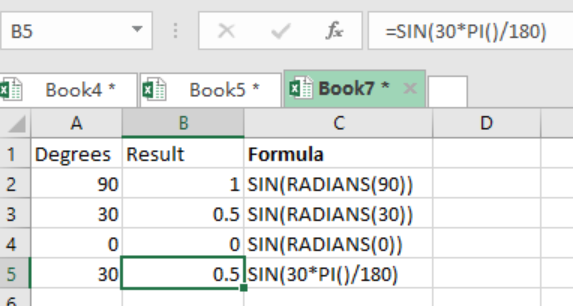Get instant live expert help with Excel or Google Sheets“My Excelchat expert helped me in less than 20 minutes, saving me what would have been 5 hours of work!”

#### Post your problem and you’ll get Expert help in seconds.

Your message must be at least 40 characters
Our professional Expert are available now. Your privacy is guaranteed.

# Learn how to Find the Sine of an Angle in Excel

Are you struggling with the concept of sine of an angle? Well, this might be quite a hustle for most of us. But it is quite easy to find the sine of any angle. This post will provide a clear way of how to find the sine of an angle in excel.Figure 1. Final result

## General syntax of the formula

`=SIN(value)`

Note that the value argument refers to the angle, which is expressed in radians.

`=RADIANS(degrees)`

## How the formula works

• To get the sine of an angle in excel, we need to understand to use the SIN function as well as the RADIANS functions.
• The RADIANS function is important due to the fact that the SIN function does not expect angles in degrees, but in radians instead.
• The formula below can be used to combine the SIN and RADIANS functions for ease of finding the sine of an angle;
`=SIN(RADIANS(value))`

## Instant Connection to an Expert through our Excelchat Service

Most of the time, the problem you will need to solve will be more complex than a simple application of a formula or function. If you want to save hours of research and frustration, try our live Excelchat service! Our Excel Experts are available 24/7 to answer any Excel question you may have. We guarantee a connection within 30 seconds and a customized solution within 20 minutes.

Solution examplesIf I merged a multi-row, multi-column selection. Iand types some text into the merged cell and then unmerges it, what will happen to the text?
Solved by S. Y. in 60 minsIF SUM OF RANGE IS MORE THAN ZERO,THEN SUM IF THE RANGE IS BLANK THEN BLANK IF THE RANGE HAS ONLY ZERO,THEN ZERO HOW SHALL I COMBINE THE ABOVE THREE IN A SINGLE CELL??
Solved by M. D. in 18 minsi need a formula to calculate previous day intraday high from google finance to google sheet. I AM USING THIS ONE =GOOGLEFINANCE(A2,"high",today()-1) BUT ITS NOT WORKING AND GIVING ME ERROR "When evaluating GOOGLEFINANCE, the query for the symbol: 'HDFCBANK' returned no data." HERE A2 IS "HDFCBANK" A TICKER.. AND I ALSO DONT WANT DATE COLUMN WITH THE PREVIOUS DAY INTRADAY HIGH PRICE.
Solved by G. E. in 40 mins